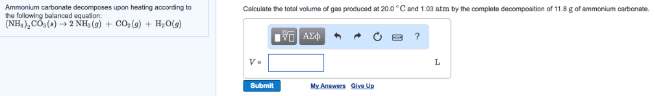### Learn how to solve problems like this one with our expert tutor.

🤓 Based on our data, we think this question is relevant for Professor Finnegan's class at WSU.

# Solution: Ammonium carbonate decomposes upon heating according to the following balanced equation: (NH4)2CO3 (s) → 2 NH3 (g) + CO2 (g) + H2O (g) Calculate the total volume of gas produced at 20.0°C and 1.03 atm by the complete decomposition of 11.8 g of ammonium carbonate.

###### Problem

Ammonium carbonate decomposes upon heating according to the following balanced equation:

(NH4)2CO3 (s) → 2 NH3 (g) + CO2 (g) + H2O (g)

Calculate the total volume of gas produced at 20.0°C and 1.03 atm by the complete decomposition of 11.8 g of ammonium carbonate.View Complete Written Solution